# Relations between Bessel functions

The relationships between the various kinds of Bessel functions are summarized in the diagram below.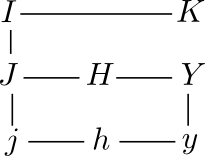The functions in the left column are functions “of the first kind” and the functions in the right column are functions “of the second kind.” The functions in the middle column are “Hankel functions.” The functions in each row satisfy a particular differential equations. The lines between functions represent simple relationships. Details are given below.

## Basic Bessel functions

The most basic Bessel functions are the functions Jν(z) imaginatively named “Bessel functions of the first kind.” I won’t go into their definition here, but I’ll explain how all the other functions in the Bessel function family reduce to these functions. For definitions and numerous identities, see functions.wolfram.com.

The functions Yν(z) are called the “Bessel functions of the second kind.” They are linear combinations of Jν and J: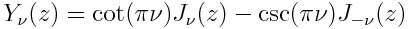Why define the functions Yν? They Yν functions are independent solutions to the differential equation that motivated the functions Jν. Specifically, Jν and Yν form a basis for the solutions to Bessel’s equation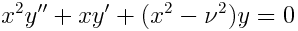In general, functions “of the second kind” were created to form independent solutions to a differential equation satisfied by the corresponding function of the first kind.

## Hankel functions

The Hankel functions are linear combinations of the Bessel functions of the first and second kind.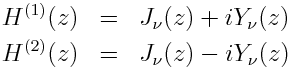## Spherical Bessel functions

Next we come to the spherical Bessel functions, so named because they arise when solving the Helmholtz equation in spherical coordinates.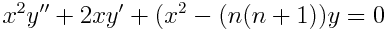For integers n, the functions jn and yn are related to the functions Jn + ½ and Yn + ½.## Spherical Hankel functions

The spherical Bessel functions have their Hankel function counterparts h(1)n and h(2)n. These are formed from jn and yn exactly the same way H(1)n and H(2)n are formed from Jn and Yn.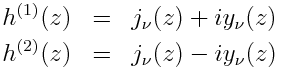## Modified Bessel functions

Finally we have the functions Iν and Kν, the “modified Bessel functions of the first and second kind.” Iν is essentially Jν with its argument rotated. Specifically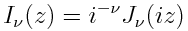Also, the functions Kν are related to the functions Iν analogously to the way the Yν are related to the Jν, namely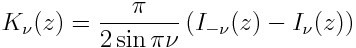The functions Iν and Kν are independent solutions to the modified Bessel differential equationThe modified Bessel functions of the second kind were also known by several names that are seldom used anymore.

• Basset functions
• Modified Bessel functions of the 3rd kind
• Modified Hankel functions
• Macdonald’s functions

The diagram would be symmetric if Kν were as simply related to Yν as Iν is related to Jν. Unfortunately, that is not the case.

## Other diagrams on this site

See this page for more diagrams on this site including diagrams for probability and statistics, analysis, topology, and category theory.